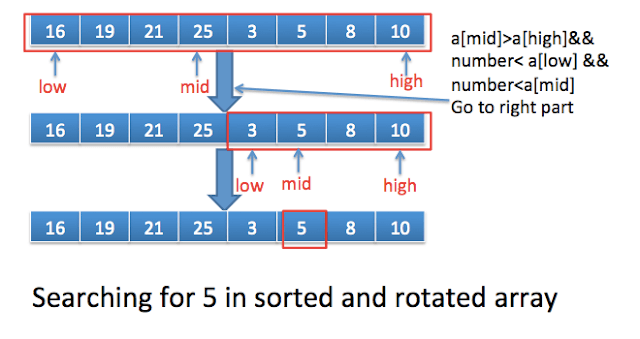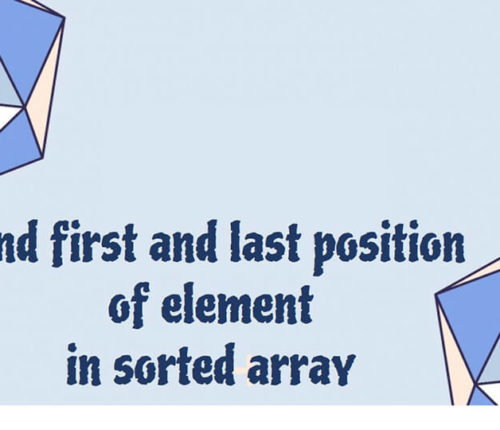# search an element in a sorted and rotated array in java

If you want to practice data structure and algorithm programs, you can go through data structure and algorithm interview questions.

In this post, we will see how to search an element in sorted and rotated array.

#### Problem:

You are given an sorted and rotated array as below:

If you note that array is sorted and rotated. You need to search an element in above array in o(log n) time complexity.

#### Solution:

You can search an element in above array using linear search but that will take o(n).
You can use variant of binary search algorithm to solve above problem. You can use a property that you can divide array into two sorted sub arrays ({16,19,21,25},{3,5,8,10} ), although you do not need to find pivot point(Elements start decreasing).

#### Algorithm:

• Compute mid i.e low+high/2.
• Check if a[mid…high] is sorted
• If number lies between the range , low=mid+1.
• If number does not lie in the range, high=mid-1.
• Check if a[low..mid] is sorted.
• If number lies between the range, high=mid-1..
• If number does not lie in the range,low=mid+1.

Lets understand this with the help of example.Steps involved to search 5 in above array:

• Compute mid i.e. 3 (0+7/2)
• a[mid](25)  >  a[high](10) && 5 < a[low](16) && 5< a[high] (25), so number (5) lies  in right part, so low will become mid+1.
• a[mid] ==5, so you can return it.

#### Java program to search an element in a sorted and rotated array :

Create a class named
SearchElementSortedAndRotatedArrayMain.java.
When you run above program, you will get below output:

import_contacts

### You may also like:## Related Posts

•04 June

### Search for a range Leetcode – Find first and last position of element in sorted array

Table of ContentsApproach 1 (Using Linear Search)Approach 2 (Using Modified Binary Search-Optimal) In this article, we will look into an interesting problem asked in Coding Interviews related to Searching Algorithms. The problem is: Given a Sorted Array, we need to find the first and last position of an element in Sorted array. This problem is […]

•28 March

### Sort an array of 0s, 1s and 2s

Table of ContentsProblemSolution If you want to practice data structure and algorithm programs, you can go through Java coding interview questions. In this post, we will see how to sort an array of 0s, 1s and 2s.We have already seen a post on sort 0s and 1s in an array. Problem Given an array containing zeroes, […]

•04 March

### Check if it is possible to reach end of given Array by Jumping

Table of ContentsProblemSolution If you want to practice data structure and algorithm programs, you can go through Java coding interview questions. ﻿ ﻿ Problem Given an array with positive integers as elements indicating the maximum length of a jump which can be made from any position in the array. Check if it is possible to have […]

•17 February

### Check if Array Elements are Consecutive

Table of ContentsProblemSolutionProgram to check if Array Elements are Consecutive If you want to practice data structure and algorithm programs, you can go through 100+ data structure and algorithm programs. In this post, we will see how to check if array elements are consecutive. Problem Given an array, we need to check if array contains […]

•01 November

### Find the local minima in array

Table of ContentsProblemSolutionNaive approachEfficient approach If you want to practice data structure and algorithm programs, you can go through Java coding interview questions. In this post, we will see how to find the local minima in the array. Problem An element is local minima if it is less than its neighbors. int [] arr = {10, […]

•22 October

### Sliding Window Maximum in java

Table of ContentsProblemSolution If you want to practice data structure and algorithm programs, you can go through Java coding interview questions. In this post, we will see about Sliding Window Maximum in java Problem Given an Array of integers and an Integer k, Find the maximum element of from all the contiguous subarrays of size K. […]

## Subscribe to our newletter

Get quality tutorials to your inbox. Subscribe now.